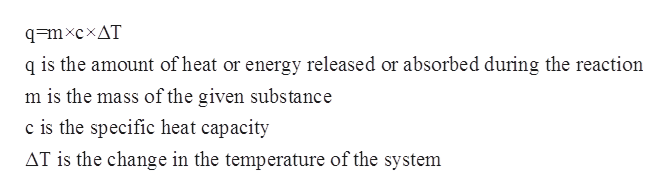# How much heat is needed to heat up 36 kg of hydrogen gas from 12.0C to 48.0C?

Question
2 views

How much heat is needed to heat up 36 kg of hydrogen gas from 12.0C to 48.0C?

check_circle

Step 1

The specific heat capacity for a given chemical substance or a solution refers to the amount of the heat that is required to increase the temperature of a given substance or system per unit of its mass. The mathematical relationship between specific heat capacity, mass, change in temperature and the heat of the solution is given by the following equation:help_outlineImage Transcriptioncloseq=mxcxAT q is the amount of heat or energy released or absorbed during the reaction m is the mass of the given substance c is the specific heat capacity AT is the change in the temperature of the system fullscreen
Step 2

The initial temperature is given to be 12 oC=285.15K.

The final temperature is given to be 48oC=321.15 K.

The mass of hydrogen gas is given to be 36 kg=36000 g.

The specific heat capacity ...

### Want to see the full answer?

See Solution

#### Want to see this answer and more?

Solutions are written by subject experts who are available 24/7. Questions are typically answered within 1 hour.*

See Solution
*Response times may vary by subject and question.
Tagged in

### Physical Chemistry